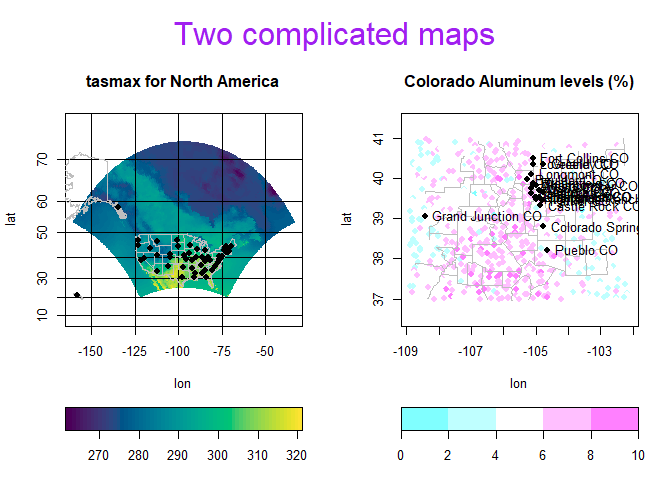Heated scatterplots

Introduction

The autoimage package makes it easy to plot a sequence of images with corresponding color scales, i.e., a sequence of heat maps, with straightforward, native options for projection of geographical coordinates. The package makes it simple to add lines, points, and other features to the images, even when the coordinates are projected.

The package also makes it easy to create a “heated scatterplot”, which is similar to a heat map in that color is used to distinguish smaller and larger values of a variable observed over a two-dimensional domain, but is different from a heat map in that the observed data locations are not gridded. Instead of interpolating the data locations onto a grid, the colors are displayed through a typical two-dimensional scatterplot.

The autopoints function is the main function for creating a sequence of heated scatterplots; it relies on the helper function heat_ppoints, which can be used in more complex scenarios. We provide the examples below with brief explanation.

autopoints Examples

We provide examples using the non-gridded Aluminum and Cadmium measurements for the state of Colorado, which are available in the gear package.

Here is a basic heated scatterplot for Aluminum with custom x and y axis labels.

library(autoimage)
data(co, package = "gear")
autopoints(co$longitude, co$latitude, co$Al, xlab = "lon", ylab = "lat")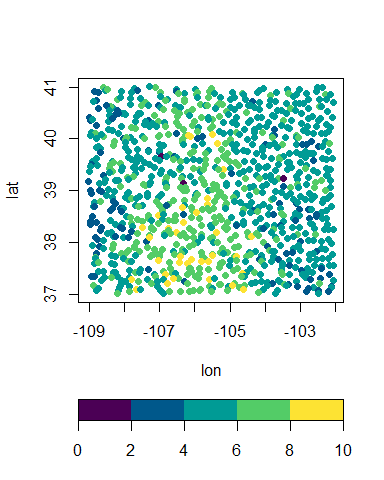In the sets of examples, we display heated scatterplots for Aluminum and Cadmium while allowing them to have different z limits and z limit breaks for the color scale. We illustrate a few different combinations using defaults and by specifying zlim and breaks as lists. The following example uses the autopoints default options, which automatically determines the color scale break labels using the the base pretty function. autopoints(co$longitude, co$latitude, co[, c("Al", "Ca")], xlab = "lon", ylab = "lat", common.legend = FALSE, main = c("Al", "Ca"))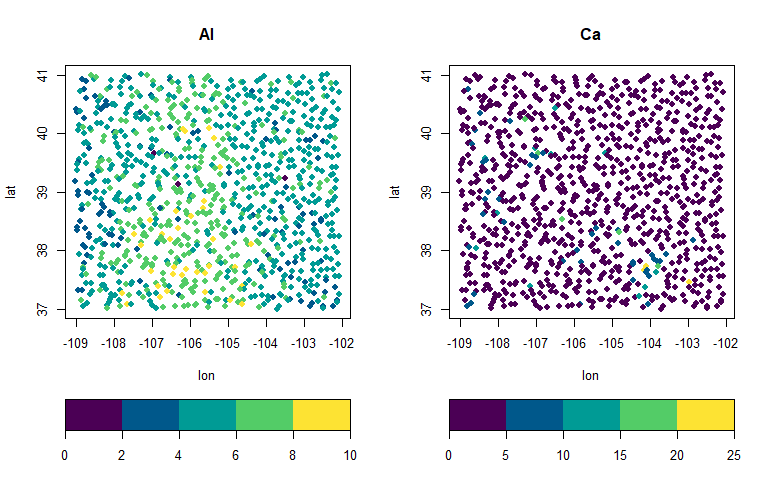In the next example, we manually specify the z limits, after using the summary function to determine appropriate limis. summary(co[, c("Al", "Ca")]) ## Al Ca ## Min. :1.120 Min. : 0.110 ## 1st Qu.:4.660 1st Qu.: 0.710 ## Median :5.540 Median : 1.230 ## Mean :5.531 Mean : 1.963 ## 3rd Qu.:6.353 3rd Qu.: 2.375 ## Max. :9.980 Max. :21.900 autopoints(co$longitude, co$latitude, co[, c("Al", "Ca")], xlab = "lon", ylab = "lat", common.legend = FALSE, main = c("Al", "Ca"), zlim = list(c(0, 10), c(0, 22)))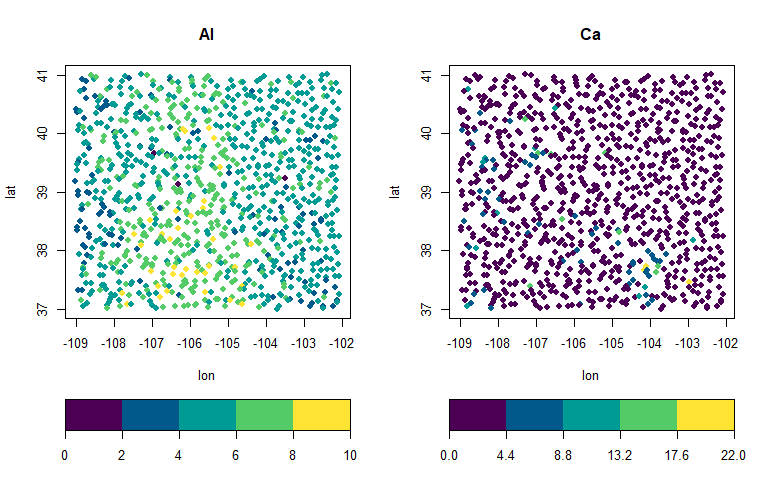Finally, we manually specify the breaks of each variable, which allows for different numbers of colors for each plot. autopoints(co$longitude, co$latitude, co[, c("Al", "Ca")], xlab = "lon", ylab = "lat", common.legend = FALSE, main = c("Al", "Ca"), breaks = list(seq(0, 10, by = 2.5), c(0, 2, 4, 6, 8, 22)))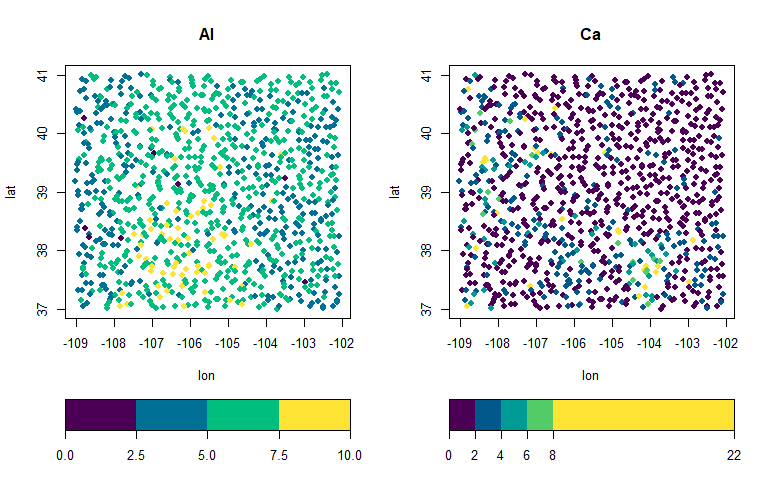In the following example, we project the coordinates using the Bonne projection. This automatically adds longitude/latitude lines (graticules), which we lighten dramatically through the col argument of the paxes.args list. The graticules do not extend far enough by default, so we extend them further through the axis.args via the xat and yat arguments. We also add text above the Aluminum and Cadmium plots via the main argument and a common title via the outer.title argument. The mtext.args argument list can be used to customize the outer title. autopoints(co$longitude, co$latitude, co[, c("Al", "Ca")], xlab = "lon", ylab = "lat", proj = "bonne", parameters = 40, paxes.args = list(col = "lightgrey"), axis.args = list(yat = 35:43, xat = -(110:101)), main = c("(a) Aluminum", "(b) Cadmium"), outer.title = "Geochemical measurements", mtext.args = list(col = "blue", cex = 2)) ## Warning in autolayout(size, legend = legend, common.legend = common.legend, : There is no room in the outer margin for an outer title. ## Setting par(oma = c(0, 0, 3, 0)).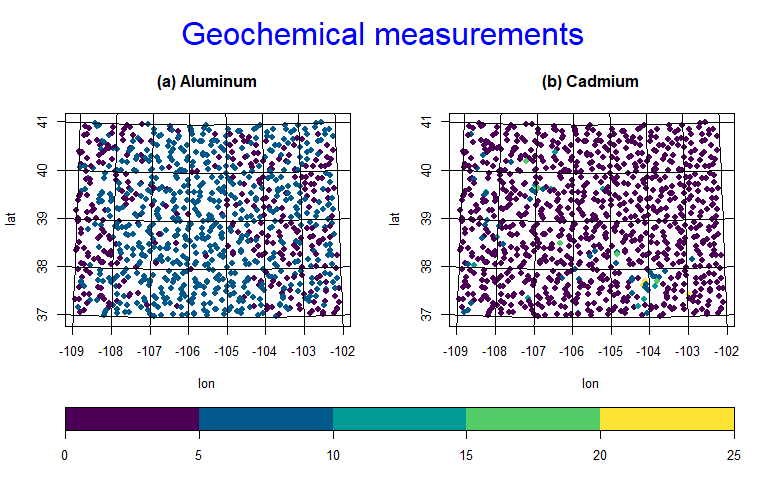We can add selected borders via the map arguments (which makes use of the the maps::map function. We can also add borders/lines via the lines arguments. We demnstrate using USA county maps (map = "county") and Colorado state borders (lines = copoly) where copoly is a polygon list available in the autoimage package. The lines can be customized through the lines.args argument list. autopoints(co$longitude, co$latitude, co$Al,
xlab = "lon", ylab = "lat", map = "county",
lines.args = list(col = "magenta"))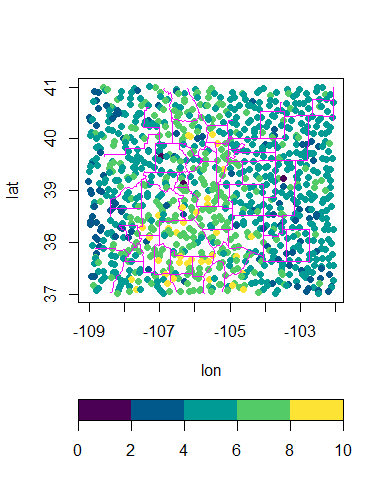data(copoly)
autopoints(co$longitude, co$latitude, co$Al, xlab = "lon", ylab = "lat", lines = copoly, lines.args = list(col = "orange", lwd = 3))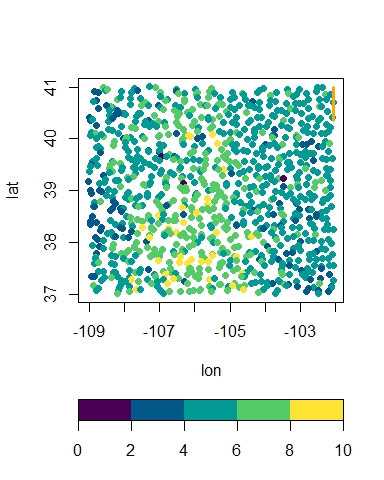Naturally, one may wish to change the color palette or the breaks associated with the colors. This can be done via the col and breaks arguments. breaks must be a sequential vector with length one element longer than col. We also move the legend scale to the right side. autopoints(co$longitude, co$latitude, co$Al, legend = "v",
xlab = "lon", ylab = "lat",
col = colorspace::sequential_hcl(n = 4, palette = "Plasma"),
breaks = c(0, 1, 2, 3, 8))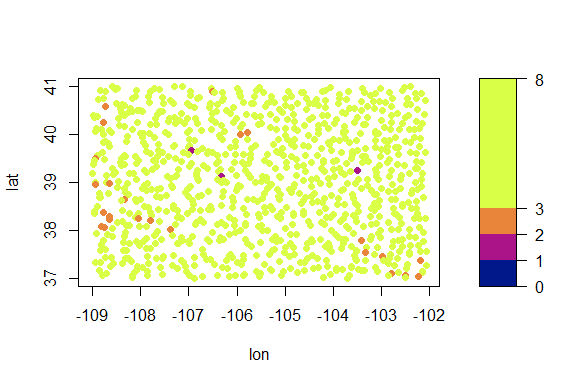We add the locations and names of two Colorado cities to the Colorado geochemical data using the points and text arguments. The appearance of these can be customized through the points.args and text.args argument lists, respectively.

citypoints = list(x = c(-104.98, -104.80), y = c(39.74, 38.85),
autopoints(co$lon, co$lat, co[,c("Al", "Ca")], common.legend = FALSE,
points = citypoints,
points.args = list(pch = 6, col = "magenta"),
text = citypoints,
text.args = list(pos = 3, col = "orange"),
xlab = "lon", ylab = "lat")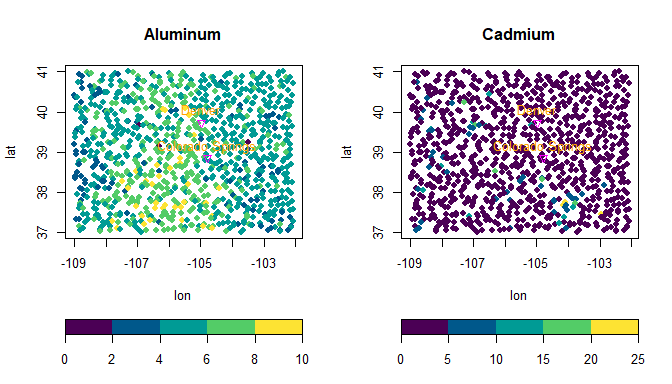Richer plots using autolayout and autolegend

Suppose we want to add custom features to a sequences of images and heated scatterplots, with each plots receiving different features. One can create a richer sequence of images using the autolayout and autolegend functions.

The autolayout function partitions the graphic device into the sections needed to create a sequence of images. The most important function arguments include size, legend, common.legend, and lratio, which correspond to the same arguments in the autoimage function. The outer argument specifies whether an outer.title is desired. The default is FALSE. By default, numbers identify the plotting order of the sections, though these can be hidden by setting show = FALSE. As an initial example, we create a 2 $$\times$$ 3 grid of images with a common vertical legend.

We now create a complicated (though unrealistic) example of this. We first extract the borders of Hawaii and Alaska from the "world" map in the maps package and store it as the hiak list. We then select a small subset of cities in Colorado from the us.cities dataset in the maps package and store this in the codf data frame. Lastly, we select the U.S. capitals from the us.cities dataset and store this in the capdf data frame.

data(worldMapEnv, package = "maps")
# extract hawaii and alaskan borders
plot = FALSE)
data(us.cities, package = "maps")
# extract colorado cities from us.cities
codf <- us.cities[us.cities$country.etc == "CO", ] # select smaller subset of colorado cities # extract capitals from us.cities capdf <- us.cities[us.cities$capital == 2,]

Having obtained the relevant information, we setup a 1 $$\times$$ 2 matrix of images with individual horizontal legends and an area for a common title. We create an image plot of NARCCAP data using the mercator projection and including grey state borders. The borders of Hawaii and Alaska are added using the plines function. The state capitals are added to the image using the ppoints function. The first image is then titled using the title function. The legend is then added using the autolegend function. Next, a heated scatterplots of the Colorado Aluminum measurements is created using the heat_ppoints function. The coordinates are projected using the Bonne projection, the color scheme is customized, grey county borders are added to the plot, but the grid lines are removed. The ppoints function is then used to add locations for several Colorado cities to the image. The ptext function is then used to add the names of these cities to the image. The second heated scatterplot is then titled using the title function. The appropriate legend is then added using the autolegend function. Lastly, a common title is added using the mtext function.

# setup plotting area
autolayout(c(1, 2), legend = "h", common.legend = FALSE, outer = TRUE)
## Warning in autolayout(c(1, 2), legend = "h", common.legend = FALSE, outer = TRUE): There is no room in the outer margin for an outer title.
##               Setting par(oma = c(0, 0, 3, 0)).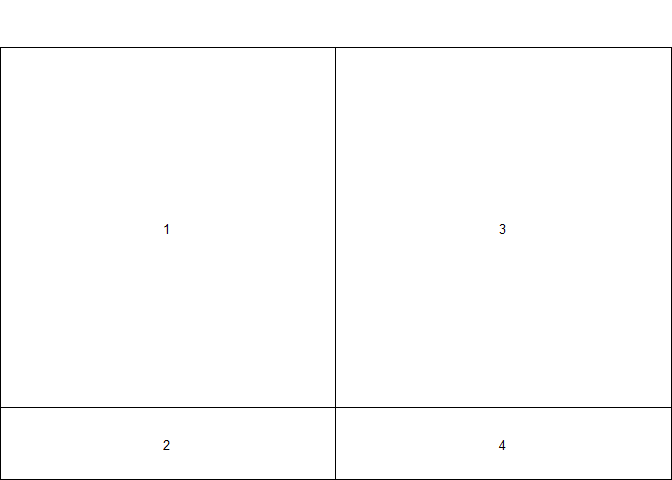# create image of NARCCAP data.
# xlim is chosen so to include alaska and hawaii
# extend graticules (longitude/latitude grid lines)
pimage(lon, lat, tasmax[,,1], legend = "none",
proj = "mercator", map = "state",
xlim = c(-180, 20),
axis.args = list(xat = seq(-175, -25, by = 25),
yat = seq(-10, 80, len = 10)),
lines.args = list(col = "grey"))
plines(hiak, proj = "mercator", col = "grey")
# add state captials to image
ppoints(capdf$lon, capdf$lat, proj = "mercator", pch = 16)
# title image
title("tasmax for North America")
autolegend()
data(co, package = "gear")
# create image for colorado aluminum measurements
# use bonne projection
# customize legend colors
heat_ppoints(co$lon, co$lat, co$Al, map = "county", legend = "none", proj = "bonne", parameters = 39, paxes.args = list(grid = FALSE), col = cm.colors(5), lines.args = list(col = "grey"), xlab = "lon", ylab = "lat") # add colorado city points to image ppoints(codf$lon, codf$lat, pch = 16, proj = "bonne") # add names of colorado cities to image ptext(codf$lon, codf$lat, labels = codf$name, proj = "bonne", pos = 4)